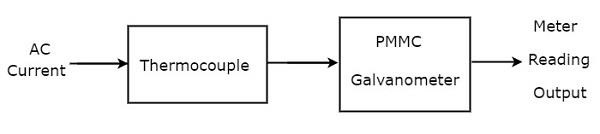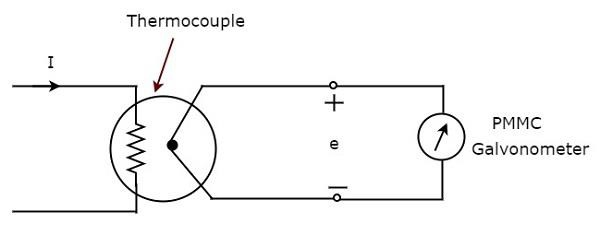# AC Ammeter

Current is the rate of flow of electric charge. If the direction of this electric charge changes regularly, then the resultant current is called Alternating Current (AC).

The instrument, which is used to measure the Alternating Current that flows through any branch of electric circuit is called AC ammeter.

Example − Thermocouple type AC ammeter.

Now, let us discuss about Thermocouple type AC ammeter.

## Thermocouple Type AC Ammeter

If a Thermocouple is connected ahead of PMMC galvanometer, then that entire combination is called thermocouple type AC ammeter. The block diagram of thermocouple type AC ammeter is shown in below figure.The above block diagram consists of mainly two blocks: a thermocouple, and a PMMC galvanometer. We will get the corresponding circuit diagram, just by replacing each block with the respective component(s) in above block diagram. So, the circuit diagram of thermocouple type AC ammeter will look like as shown in below figure.Thermocouple generates an EMF, $e$, whenever the Alternating Current, I flows through heater element. This EMF, $e$ is directly proportional to the rms value of the current, I that is flowing through heater element. So, we have to calibrate the scale of PMMC instrument to read rms values of current.

So, with this chapter we have completed all basic measuring instruments such as DC voltmeters, AC voltmeters, DC ammeters and AC ammeters. In next chapter, let us discuss about the meters or measuring instruments, which measure resistance value.